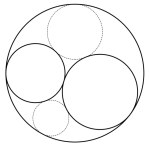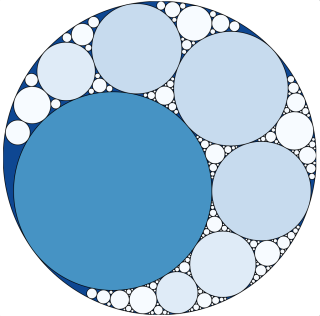by Jonathan Kujawa

Apollonius of Perga (262-190 BC) was a well known and prolific geometer in ancient Greece. He is mainly known for his surviving work on the conic sections. Indeed, he gave us the definition of the ellipse, parabola, and hyperbola we use today. In some circles, Apollonius's most famous theorem is the fact that if you have three circles which are mutually tangent, then there always exactly two ways to add a fourth circle which is tangent to all three. That is, you can always fit exactly one new circle tangent to the original three within each interstice made by the existing circles. In the picture to the right if you start with the three black circles, then you can complete the picture to four tangent circles by adding either of the gray circles.

One thing I've learned in math is: anything worth doing once, is worth doing many times. Once you add the two new circles you've now created six new gaps. This, in turn, can be filled with circles, creating yet more gaps. And so on. You can keep adding circles forever:Image from .

The fractally looking result is an Apollonian Gasket. There is a delightful Gasket maker available here. You get fun pictures like this one:As we did above, it is common when drawing Gaskets to start with two circles inside of a third, but this is only for convenience and isn't needed for Apollonius's result. Indeed, if you start with three circles which are tangent to each other and none contains the others, then one of the two new circles you add will encircle the others, so you'll end up with the outside circle, anyway.

When looking at pictures like these, it is natural to ask how much of the big circle is filled by the smaller circles. At each stage there will always be little slivers of empty space. But perhaps eventually every gap is filled with a circle. If you know about fractals, you'll know the answer to this question is not so obvious.

It could be the circles fill the big circle completely, or they could leave sizable gaps, or it could be somewhere in between. When dealing with fractals, the correct measure of size is something called the Hausdorff dimension. As it should be, a line, square, and cube have Hausdorff dimension 1, 2, and 3, respectively. However, it is entirely possible for the Hausdorff dimension to be somewhere in between. Our intuition is if you thicken a line by even the tiniest bit, you now have a two-dimensional rectangle. But the universe is much more interesting than our imaginations allow . It is entirely possible for a shape to have Hausdorff dimension 1.5. Somehow a shape can be thicker than a line, but not quite as wide as a rectangle!

Indeed, in the 1990s Curtis McMullen computed the Hausdorff dimension of the region made by the gaps of an Apollonian Gasket and showed that it is approximately 1.30568. This settles the question since it is true (but not obvious!) that every Apollonian Gasket has the same Hausdorff dimension. By way of comparison, the fractal dimension of the west coast of Britain is approximately 1.25; the inside of an Apollonian Gasket is only slightly thicker than the coastline of Britain.

In the 1640s Descartes exchanged a series of letters with Princess Elizabeth of Bohemia on philosophy and mathematics. No doubt aware of Appolonius's theorem, Descartes posed to Princess Elizabeth the Problem of the Three Circles: given three circles prove there is always a circle tangent to all three and describe that circle. Out of their correspondence, Descartes made a remarkable discovery.

To explain it, we first need one new concept. If a circle has radius r, then the curvature of the circle is the number 1/r. It is a measure of how sharply you would have to turn if you drove around the circle. The smaller the radius, the smaller the circle, and the sharper the curve of the circle (and the larger the curvature). The larger the radius, the larger the circle, and the closer to straight the circle's curve (and the smaller the curvature).

If you start with three mutually tangent circles which have curvatures a, b, and c, and if the fourth tangent circle guaranteed by Appolonius's theorem has curvature d, then Descartes proved the four curvatures satisfy the equation:This remarkable formula is now known as Descartes's quadratic equation . This relationship between curvatures was rediscovered numerous times over the years. in the 1930s the Nobel laureate Frederick Soddy (re)discovered the formula yet again. He was so pleased, he wrote a poem for the journal Nature. Here's the relevant stanza (emphasis added):

Four circles to the kissing come.
The smaller are the benter.
The bend is just the inverse of
The distance form the center.
Though their intrigue left Euclid dumb
There's now no need for rule of thumb.
Since zero bend's a dead straight line
And concave bends have minus sign,
The sum of the squares of all four bends
Is half the square of their sum.

It probably goes without saying, but Soddy's Nobel prize wasn't for literature. While the equation itself wasn't new, Soddy did notice a surprising new consequence of Descartes's quadratic equation: if the curvatures of four of our tangent circles happen to be integers (i.e. 1,2,3,4,…), then so must be the curvature of the fifth circle guaranteed by Appolonius's theorem.

Soddy's observation means a truly amazing fact is true: when making a Gasket, if the first few circles have integral curvatures, then every circle in the Gasket has an integral curvature! Such Gaskets are called integral Apollonian gaskets, and we'll focus on them from now on. Here is an integral Gasket from Wikipedia with the curvature of each circle written in:Number theory (the study of the integers) is as old as the Greeks. It is rather surprising only a handful of mathematicians over the decades bothered to explore the numbers which could occur as curvatures in Apollonian Gaskets. As far as I know, the field was really launched in a series of rather recent papers by Graham, Lagarias, Mallows, Wilks, and Yan (GLMWY). They showed these Apollonian numbers have numerous interesting properties and conjectured many more based upon their computer calculations.

Verifying one of their conjectures, Peter Sarnak showed in 2007 that the collection of curvatures for an Apollonian Gasket always contains an infinite number of primes. He went further and proved an Apollonian Gasket always contains an infinite number of pairs of tangent circles who both have prime curvatures! Sarnak also proved every Apollonian Gasket contains arbitrarily long chains of circles, each tangent to the next, which have prime curvatures.

In a different direction, you could ask how "dense" the curvatures are among the collection of all integers. Since the circles in a Gasket become ever smaller, their curvatures ever increase. This means we know there are infinitely many different curvatures, but it is entirely possible for them to become sparser and sparser. For example, there are infinitely many perfect squares (1, 4, 9, 16, 25, …), but they spread further and further out as you go and are vanishingly rare in the sea of integers.

What about the curvatures of the circles in a Gasket? GLMWY proved that, unlike the perfect squares, the curvatures of a Gasket have a nontrivial density in the integers. To be more precise, they proved the number of integers less than n which occur as the curvatures of a Gasket is more than √n .

Doing much better, in 2011 Jean Bourgain and Elena Fuchs proved the number of curvatures in a Gasket less than n is a constant fraction of n. That is, for any given integral Gasket, nearly all integers occur as curvatures.

Like Hercules and the Hydra, every advance in our mathematical understanding raises even more questions. The famous Green-Tao theorem tells us the prime numbers contain arbitrarily long arithmetical progressions. A key step depends on the fact the primes are "dense" in the integers in a suitable sense. I'm no expert, but given the GLMWY and Bourgain-Fuchs results, it wouldn't at all surprise me if the curvatures of a Gasket also contain arbitrarily long arithmetic progressions. I would love to hear if this is known or not. My guess is that it is already known to be true by the experts.

One thing which is definitely not known is something called the "local-to-global conjecture". When looking at integers you can consider them "modulo 24". This means you treat them as if they are on a 24-hour clock (so 13, 37, and 61 are all equal modulo 24). Given a Gasket, Fuchs recently showed the curvatures only give you a handful of the possible 24 values on the clock. For each of those values, there are infinitely many possible curvatures which give that value (e.g. the value 13 gives us 13, 37, 61, 85,…). The local-to-global conjecture says that for each of those values, all but finitely many of the possible curvatures which have that value actually occur in the Gasket.

That is, with finitely many exceptions, we think we know exactly which numbers occur as the curvatures in a Gasket. All our computer calculations and mathematical results support the conjecture, but nobody knows how to prove it!

Faithful readers will recall we discussed the problem of sphere packing here at 3QD. We saw there in the work of Viazovska that there are close connections between the geometry of circles and spheres, and number theory. So perhaps, in retrospect, it's not so surprising that the curvatures of Gaskets are so darn interesting!

In a pleasing coincidence, Robert Langlands recently received the 2018 Abel Prize. He was the first to outline a deep web of connections between geometry, number theory, and algebra which we still only partially understand. Apollonian Gaskets and their curvatures are in this circle of ideas.

 This and the previous image are from a paper by Kate Stange.

 "There are more things in heaven and earth, Horatio, than are dreamt of in your philosophy. "

 In order for Descartes's quadratic equation to be true for the outermost circle of an Apollonian Gasket, we need to take its curvature to be the negative of the usual 1/radius.

 Here I really mean some fixed, positive constant times √n. For these sorts of calculations, we are mostly interested in very, very large numbers for n and so the constant can be safely ignored. We are mostly interested in how quickly a quantity grows with n, not its precise value.

 Thanks to Ella Fitzgerald for the inspiration for the title. While googling for this essay, I discovered Dana Mackenzie used the same title in an article on Apollonian Gaskets. Great minds think alike!